# Trochoid

(diff) ← Older revision | Latest revision (diff) | Newer revision → (diff)

A plane curve that is the trajectory of a pointinside or outside a circle that rolls upon another circle. A trochoid is called an epitrochoid (Fig.1a, Fig.1b) or a hypotrochoid (Fig.2a, Fig.2b), depending on whether the rolling circle has external or internal contact with the fixed circle.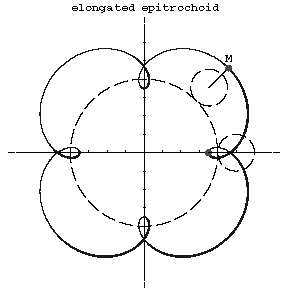Figure: t094330a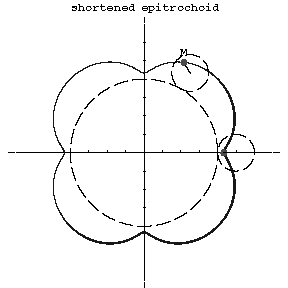Figure: t094330bFigure: t094330cFigure: t094330d

The parametric equations of the epitrochoid are: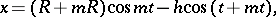and of the hypotrochoid:whereis the radius of the rolling circle,is the radius of the fixed circle,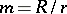is the modulus of the trochoid, andis the distance from the tracing point to the centre of the rolling circle. If, then the trochoid is called elongated (Fig.1a, Fig.2a), when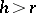shortened (Fig.1b, Fig.2b) and when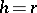, an epicycloid or hypocycloid.

If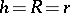, then the trochoid is called a trochoidal rosette; its equation in polar coordinates is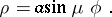For rational values ofthe trochoidal rosette is an algebraic curve. If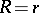, then the trochoid is called the Pascal limaçon; if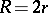, an ellipse.

Trochoids are related to the so-called cycloidal curves (cf. Cycloidal curve). Sometimes the trochoid is called a shortened or elongated cycloid.